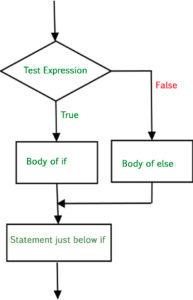# else keyword in Julia

• Last Updated : 22 Apr, 2020

Keywords in Julia are reserved words that have a specific meaning and operation to the compiler. These keywords can not be used as a variable name, doing the same will stop the execution process and an error will be raised.

‘else’ keyword in Julia is used to execute a block of code when the ‘if’ condition is false. If the condition is true, the code after the if statement will be executed otherwise the body of else statement will be executed.
Syntax:

```if condition
statement
else
statement
end
```

Flowchart:Example:

 `# Julia program to illustrate ``# the use of 'if' keyword``  ` `# function definition``function check_if(x)``      ` `    ``# if keyword``    ``if` `x > ``10``        ``println(x, ``" is greater than 10"``)``    ``else``        ``println(x, ``" is smaller than 10"``)``    ``end``    ``println(``"This is not in if"``)``end``  ` `# function call``check_if(``15``)`

Output:

```5 is smaller than 10
This is not in if
```

`'else'` keyword can also be used with `'if'` keyword to generate an ‘if-elseif-else’ ladder.

Example:

 `# Julia program to illustrate``# if-elseif-else ladder ``i ``=` `20``if` `(i ``=``=` `10``) ``    ``println(``"Value of i is 10"``) ``elseif(i ``=``=` `15``) ``    ``println(``"Value of i is 15"``) ``elseif(i ``=``=` `20``) ``    ``println(``"Value of i is 20"``) ``else``    ``println(``"Value of i is not defined"``) ``end `

Output:

```Value of i is 20
```

My Personal Notes arrow_drop_up